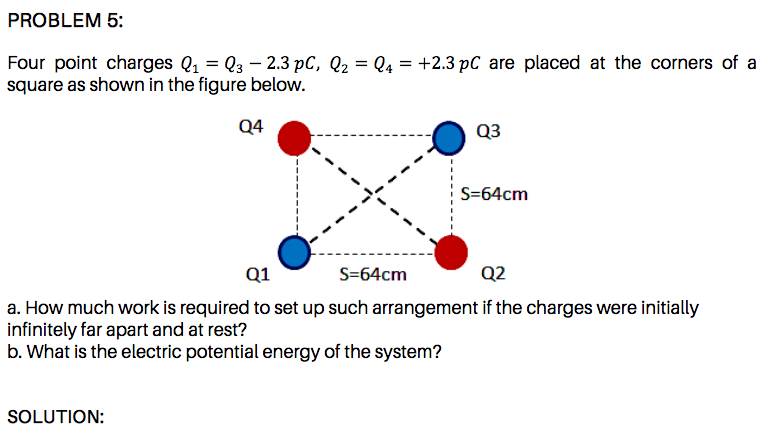# Four point charges Q1 = Q3 – 2.3 pC, Q2 = Q4 = +2.3 pC are placed at the corners of a square as shown in the figure below. Q4 Q3 S=64cm Q1 S=64cm Q2 a. How much work is required to set up such arrangement if the charges were initially infinitely far apart and at rest? b. What is the electric potential energy of the system?

Questionhelp_outlineImage TranscriptioncloseFour point charges Q1 = Q3 – 2.3 pC, Q2 = Q4 = +2.3 pC are placed at the corners of a square as shown in the figure below. Q4 Q3 S=64cm Q1 S=64cm Q2 a. How much work is required to set up such arrangement if the charges were initially infinitely far apart and at rest? b. What is the electric potential energy of the system? fullscreen

### Want to see this answer and more?

Experts are waiting 24/7 to provide step-by-step solutions in as fast as 30 minutes!*

*Response times may vary by subject and question complexity. Median response time is 34 minutes for paid subscribers and may be longer for promotional offers.
Tagged in
Science
Physics

### Electric Charges and Fields